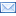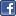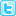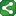## Thursday, August 21, 2008

### What to do when we want only the even number observations

Output only even number observations.

Note: The MOD function returns the remainder when values are divided. In this sample, when dividing i by 2, there will be no remainder for the even observations (2,4,6,8 and 10). If there is a remainder, the current observation has to be an odd numbered observation. This program outputs only the even numbered observations to a new data set.

/* Create sample data set */
data one;
do i=1 to 10;
output;
end;
run;
data two;
set one;

/* The MOD function returns the remainder from the division of */
/* argument-1 by argument-2. If the remainder is zero, when */
/* the second argument is 2, then the first argument must be */
/* even...therefore, output the observation. */

if mod(i,2)=0 then output;
run;

proc print;
run;

OUTPUT:
Obs i
1 2
2 4
3 6
4 8
5 10

source:www.support.sas.com

#### Post a CommentShareThis